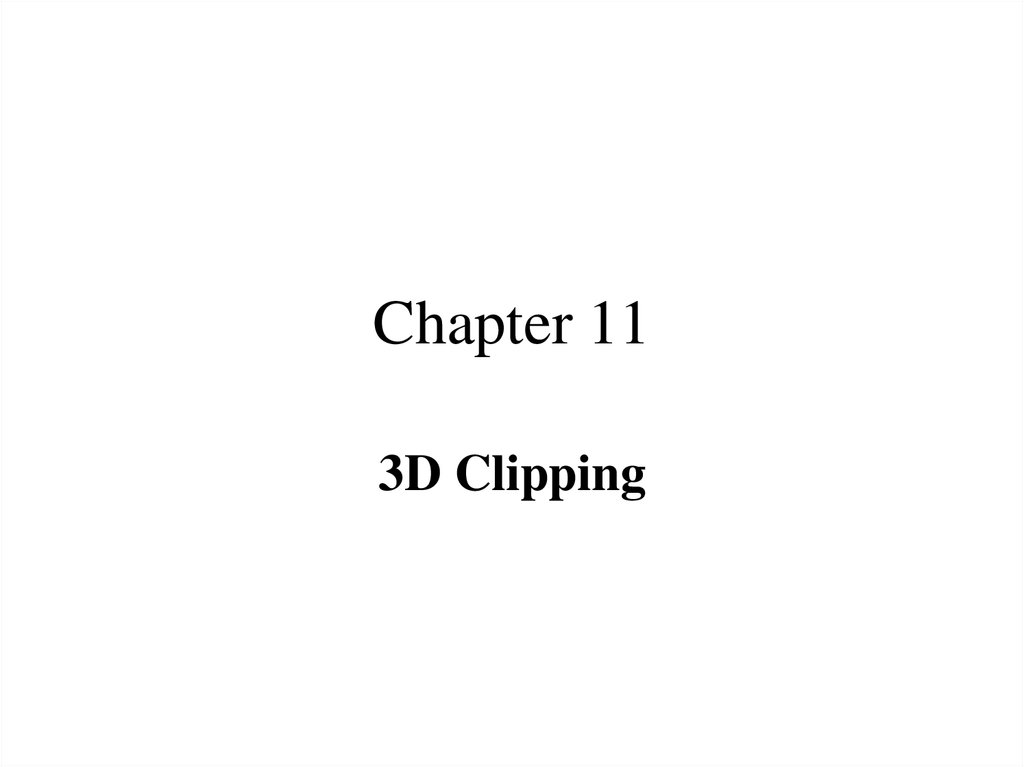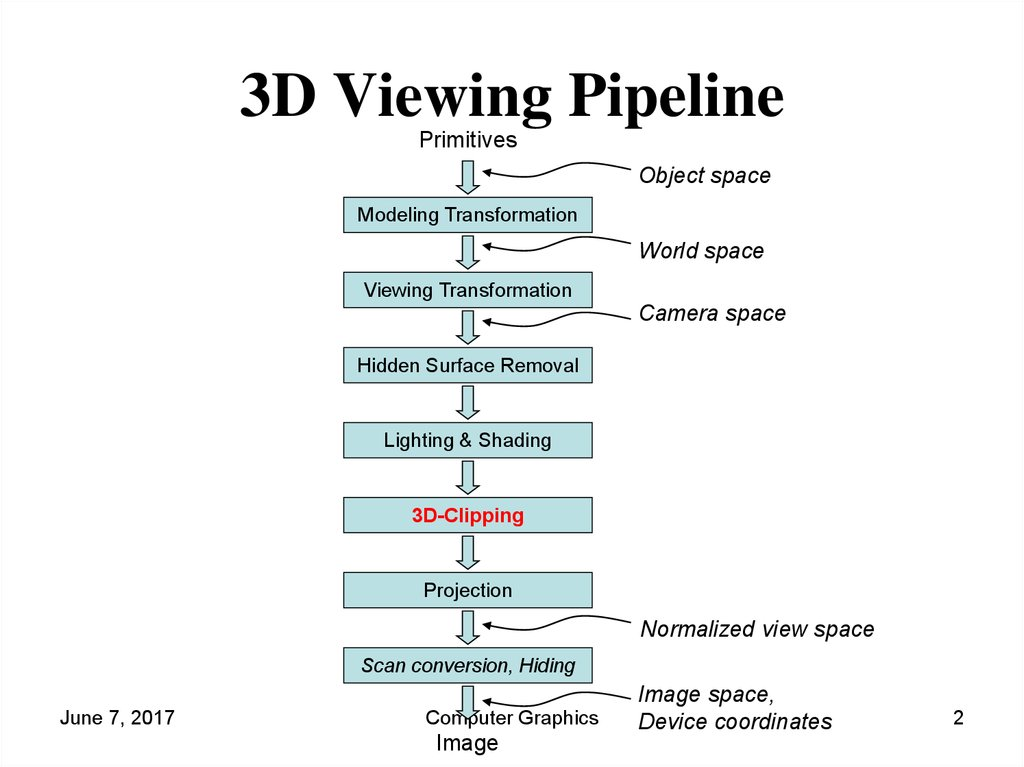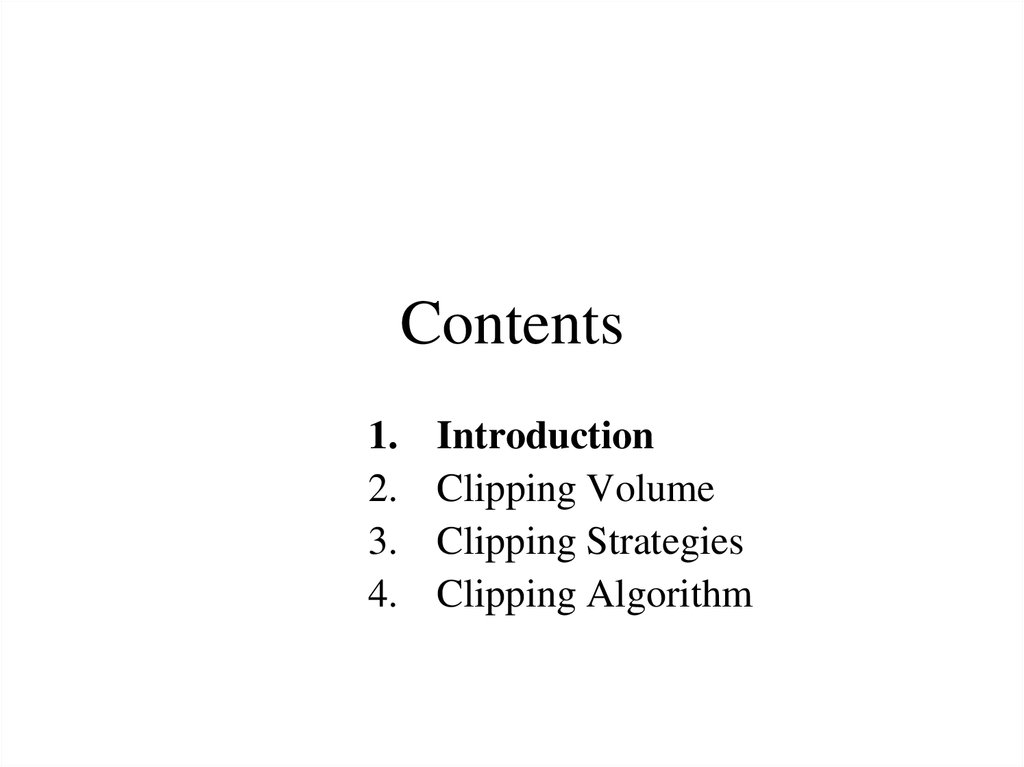# 3D Viewing Pipeline

3D Clipping

## 2. 3D Viewing Pipeline

Primitives
Object space
Modeling Transformation
World space
Viewing Transformation
Camera space
Hidden Surface Removal
3D-Clipping
Projection
Normalized view space
Scan conversion, Hiding
June 7, 2017
Computer Graphics
Image
Image space,
Device coordinates
2

## 3. Contents

1.
2.
3.
4.
Introduction
Clipping Volume
Clipping Strategies
Clipping Algorithm

## 4. 3D Clipping

• Just like the case in two dimensions, clipping removes objects
that will not be visible from the scene
• The point of this is to remove computational effort
• 3-D clipping is achieved in two basic steps
– Discard objects that can’t be viewed
• i.e. objects that are behind the camera, outside the field of view, or too far
away
– Clip objects that intersect with any clipping plane
June 7, 2017
Computer Graphics
4

## 5. 3D Clipping

• Discarding objects that cannot possibly be seen involves
comparing an objects bounding box/sphere against the
dimensions of the view volume
– Can be done before or after projection
June 7, 2017
Computer Graphics
5

## 6. 3D Clipping

• Objects that are partially within the viewing volume need to be
clipped – just like the 2D case
June 7, 2017
Computer Graphics
6

## 7. Contents

1.
2.
3.
4.
Introduction
Clipping Volume
Clipping Strategies
Clipping Algorithm

## 8. The Clipping Volume

• In case of Parallel projections the infinite Parallelepiped is
bounded by Near/front/hither and far/back/yon planes for
clipping.
June 7, 2017
Computer Graphics
8

## 9. The Clipping Volume

• In case of Perspective projections the semi Infinite Pyramid is
also bounded by Near/front/hither and far/back/yon planes for
clipping
June 7, 2017
Computer Graphics
9

## 10. The Clipping Volume

• After the perspective transformation is complete the frustum
shaped viewing volume has been converted to a parallelepiped
- remember we preserved all z coordinate depth information
June 7, 2017
Computer Graphics
10

## 11. Contents

1.
2.
3.
4.
Introduction
Clipping Volume
Clipping Strategies
Clipping Algorithm

## 12. Clipping Strategies

• Because of the extraordinary computational effort
required, two types of clipping strategies are
followed:
– Direct Clipping: The clipping is done directly against the
view volume.
– Canonical Clipping: Normalization transformations are
applied which transform the original view volume into
normalized (canonical) view volume. Clipping is then
performed against canonical view volume.
June 7, 2017
Computer Graphics
12

## 13. Clipping Strategies

• The canonical view volume for parallel projection is the unit
cube whose faces are defined by planes
x=0;x=1
y = 0; y = 1
z = 0; z = 1
June 7, 2017
Computer Graphics
13

## 14. Clipping Strategies

• The canonical view volume for perspective projection is the
truncated normalized pyramid whose faces are defined by
planes
x = z ; x = -z
y = z; y = -z
z = zf; z = 1
y
y
clipped line
clipped line
x
x
1
1
near
z
far
0
1
z
image plane
near
June 7, 2017
far
Computer Graphics
14

## 15. Clipping Strategies

• We perform clipping after the projection transformation and
normalizations are complete.
• So, we have the following:
xh
x
y
y
h M
zh
z
h
1
• We apply all clipping to these homogeneous coordinates
June 7, 2017
Computer Graphics
15

## 16. Clipping Strategies

• The basis of canonical clipping is the fact that intersection of
line segments with the faces of canonical view volume require
minimal calculations.
• For perspective views, additional clipping may be required to
avoid perspective anomalies produced by the projecting
objects that are behind view point.
June 7, 2017
Computer Graphics
16

## 17. Contents

1.
2.
3.
4.
Introduction
Clipping Volume
Clipping Strategies
Clipping Algorithm

## 18. Clipping Algorithms

3D clipping algorithms are direct adaptation of 2D clipping
algorithms with following modifications:
1.
2.
3.
4.
For Cohen Sutherland: Assignment of out codes
For Liang-Barsky: Introduction of new equations
For Sutherland Hodgeman: Inside/Out side Test
In general: Finding the intersection of Line with plane.
June 7, 2017
Computer Graphics
18

## 19. 3D Cohen-Sutherland Line Clipping

• Similar to the case in two dimensions, we divide the world into
regions
• This time we use a 6-bit region code to give us 27 different
region codes
• The bits in these regions codes are as follows:
bit 1
Above
June 7, 2017
bit 2
Below
bit 3
Right
bit 4
Left
Computer Graphics
bit 5
Behind
bit 6
Front
19

## 20. 3D Cohen-Sutherland Line Clipping

June 7, 2017
Computer Graphics
20

## 21. 3D Cohen-Sutherland Line Clipping

Now we use a 6 bit
out code to handle the
near and far plane.
The testing strategy is
virtually identical to
the 2D case.
June 7, 2017
Computer Graphics
21

## 22. 3D Cohen-Sutherland Line Clipping

CASE – I Assigning region codes to endpoints for Canonical
Parallel View Volume defined by:
x = 0 , x = 1;
y = 0, y = 1;
z = 0, z = 1
The bit codes can be set to true(1) or false(0) for depending on
the test for these equations as follows:
Bit
Bit
Bit
Bit
Bit
Bit
1
2
3
4
5
6
June 7, 2017

endpoint
endpoint
endpoint
endpoint
endpoint
endpoint
is
is
is
is
is
is
Above view volume = sign (y-1)
Below view volume = sign (-y)
Right view volume = sign (x-1)
Left view volume = sign (-x)
Behind view volume = sign (z-1)
Front view volume = sign (-z)
Computer Graphics
22

## 23. 3D Cohen-Sutherland Line Clipping

CASE – II Assigning region codes to endpoints for Canonical
Perspective View Volume defined by:
x = -z , x = z;
y = -z, y = z;
z = zf , z = 1
The bit codes can be set to true(1) or false(0) for depending on
the test for these equations as follows:
Bit
Bit
Bit
Bit
Bit
Bit
1
2
3
4
5
6
June 7, 2017

endpoint
endpoint
endpoint
endpoint
endpoint
endpoint
is
is
is
is
is
is
Above view volume = sign (y-z)
Below view volume = sign (-z-y)
Right view volume = sign (x-z)
Left view volume = sign (-z-x)
Behind view volume = sign (z-1)
Front view volume = sign (zf-z)
Computer Graphics
23

## 24. 3D Cohen-Sutherland Line Clipping

• To clip lines we first label all end points with the appropriate
region codes.
• Classify the category of the Line segment as follows
– Visible: if both end points are 000000
– Invisible: if the bitwise logical AND is not 000000
– Clipping Candidate: if the bitwise logical AND is 000000
• We can trivially accept all lines with both end-points in the
 region.
• We can trivially reject all lines whose end points share a
common bit in any position.
June 7, 2017
Computer Graphics
24

## 25. 3D Cohen-Sutherland Line Clipping

June 7, 2017
Computer Graphics
25

## 26. 3D Cohen-Sutherland Line Clipping

• For clipping equations for three dimensional line segments
are given in their parametric form.
• For a line segment with end points P1(x1h, y1h, z1h, h1) and
P2(x2h, y2h, z2h, h2) the parametric equation describing any
point on the line is:
P P1 ( P2 P1 )u
0 u 1
• From this parametric equation of a line we can generate the
equations for the homogeneous coordinates:
x h x1h ( x 2 h x1h )u
y h y1h ( y 2 h y1h )u
z h z1h ( z 2 h z1h )u
h h1 (h 2 h1)u
June 7, 2017
Computer Graphics
26

## 27. 3D Cohen-Sutherland Line Clipping

• Consider the line P1 to P2
• Because the lines have different values in bit 2 we know the line
crosses the right boundary
June 7, 2017
Computer Graphics
27

## 28. 3D Cohen-Sutherland Line Clipping

• Since the right boundary is at x = 1 we now know the following
holds:
xh x1h ( x 2h x1h )u
xp
1
h
h1 (h2 h1)u
• which we can solve for u as follows:
x1h h1
u
( x1h h1) ( x 2 h h2)
• using this value for u we can then solve for yp and zp similarly
• Then simply continue as per the two dimensional line clipping
algorithm
June 7, 2017
Computer Graphics
28